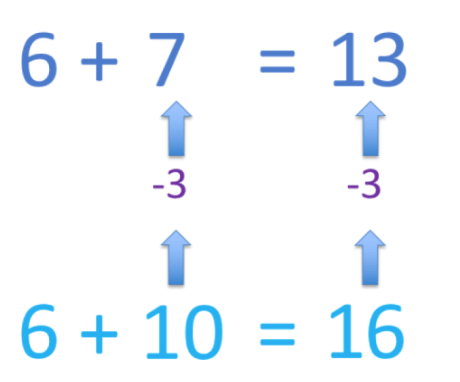# The Partial Sums Method

The Partial Sums MethodAdd each place value column separately and then add up the totals.• The partial sums method involves breaking each number down into their hundreds, tens and ones and adding them separately.
• 341 is made up of 300 + 40 + 1.
• 216 is made up of 200 + 10 + 6.
• Adding the hundreds of each number: 300 + 200 = 500.
• Adding the tens of each number: 40 + 10 = 50.
• Adding the ones of each number: 1 + 6 = 7.
• Finally, we add these results together: 500 + 50 + 7 = 557.• In 55 + 36 we have 50 + 5 and 30 + 6.
• Adding the tens: 50 + 30 = 80.
• Adding the ones: 5 + 6 = 11.
• Finally, adding these partial sums: 80 + 11 = 91.

• In 231 + 194 we have 200 + 30 + 1 and 100 + 90 + 4
• Adding the hundreds: 200 + 100 = 300.
• Adding the tens: 30 + 90 = 120.
• Adding the ones: 1 + 4 = 5.
• Finally, adding these partial sums: 300 + 120 + 5 = 425.Supporting Lessons

# The Partial Sums Method

## What is the Partial Sums Method?

The partial sums method is an addition strategy in which the numbers are added together in parts according to their place value. For example, 635 + 312 is calculated by adding 600 + 300 = 900, 30 + 10 = 40 and 5 + 2 = 7. These partial sums are then added as 900 + 40 + 7 = 947.600 + 300 = 900

30 + 10 = 40

5 + 2 = 7

900 + 40 + 7 = 947

## Partial Sums Method Examples

Here are some examples of using the partial sums method.

Addition Sum Sum of the Hundreds Sum of the Tens Sum of the Ones Adding the Partial Sums
346 + 523 300 + 500 = 800 40 + 20 = 60 6 + 3 = 9 800 + 60 + 9 = 869
105 + 382 100 + 300 = 400 0 + 80 = 80 5 + 2 = 7 400 + 80 + 7 = 487
645 + 115 600 + 100 = 700 40 + 10 = 50 5 + 5 = 10 700 + 50 + 10 = 760
361 + 155 300 + 100 = 400 60 + 50 = 110 1 + 5 = 6 400 + 110 + 6 = 516
269 + 363 200 + 300 = 500 60 + 60 = 120 9 + 3 = 12 500 + 120 + 12 = 632

## Subtraction with Partial Differences

The partial differences subtraction method involves subtracting each place value column individually and then finding the total of these results. Always subtract the smaller number from the larger number. If the first number is subtracted from the second number, the result will be negative.

Use the partial differences method to subtract 625 – 453.625 is made up of 600 + 20 + 5.

453 is made up of 400 + 50 + 3.

• Subtracting the hundreds

600 – 400 = 200

• Subtracting the tens

20 – 50 = -30.

Alternatively, 50 – 20 = 30 but since the numbers were subtracted the other way around, we write -30.

• Subtracting the ones

5 – 3 = 2

• Finding the total

200 – 30 + 2 = 172.

Therefore 625 – 453 = 172.

Here is another example of using the partial differences method of subtraction.

Calculate 814 – 366.• Subtracting the hundreds

800 – 300 = 500

• Subtracting the tens

We need to subtract the numbers in the opposite order and therefore, the result will be negative.

60 – 10 = -50.

• Subtracting the ones

Again, we need to subtract the numbers in the opposite order and therefore, the result will be negative.

6 – 4 = -2.

• Finding the total

500 – 50 – 2 = 448.

Therefore 814 – 366 = 448.Now try our lesson on Addition Using the Compensation Method where we learn how to use the compensation method for adding two numbers.error: Content is protected !!